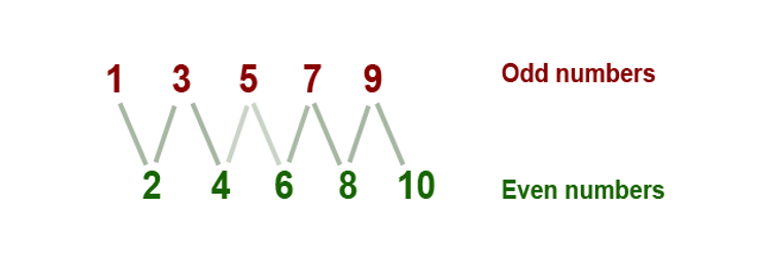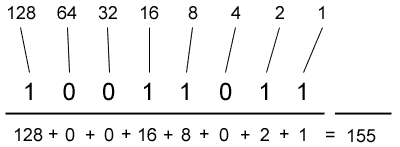# Parity Check Program in C++In this task, we need to implement a function that would check the number of parity using only the bit operations AND, OR, and NOT.

Solution

Note that the number x is odd only when the youngest (the first on the right) bit in its binary representation is equal to 1. Let us prove this. Recall the school rule of the algorithm for translation numbers from the binary system into the decimal. It is shown in the following picture:We can take 2 out of the context for all terms except the last, which can be equal to either 1 or 0. Thus, if it is zero, then the sum will be in the form 2 (…) = x, then it will be divided by 2, and if it is equal to 1, then the amount will be in the form 2 (…) +1 = x, then it will not be divided by 2. This is the criterion of parity.

So we have proved the fact that the number is odd when the least significant bit is set to 1, and even when the least significant bit is 0. The question remains: how do you get the last bit of the number? Adoption: the last bit number x is equal to x & 1, where & is the bitwise AND. Why is this so? AND is equal to 1 only if both of its arguments are equal to 1. The number 1 in the binary system is as follows: … 000001 (depending on how many bit numbers we operate). So, for the bitmap AND for 1 with the result equal to number x all the bits, except the last, will be equal to zero, and the last bit will be equal to 1 if int the number x was equal to 1 (1 & 1 = 1), and 0 if int the number x was equal to 0 (0&1 = 0).

Thus, the value of the x&1 expression is 1 if it’s an odd number, and 0 if x is an even number.

The sample about the parity check program in C that you have just examined was completed by a programmer from AssignmentShark. As a rule, we try to provide students with examples that can help them to solve difficult tasks, like our C++ regular expression example. Unfortunately, our samples are not very specific. Each task is unique and sometimes general samples can’t help you. If this is the case, you can order an original sample that will be completed just for you. Know how to do this by reading the next paragraph.

If you would like to make an order, you should fill the order form on our website. You need to specify the deadline, the discipline and the topic, as well as other details about the task. Afterward, you will submit the order form and our experts will examine it. The experts who are available at the moment and who are the most knowledgeable in the issue will offer you their prices. This option is paid because the sample will be written only for you. We won’t publish it. You’ll be the sole owner of the sample.

Our sample about a parity check program in C is only one from numerous samples that concern programming. You can look through other examples absolutely for free. We don’t establish prices for examples that are published. If you can’t find the specific sample, please, contact us! We are always ready to help with assignment writing in your trying hour!